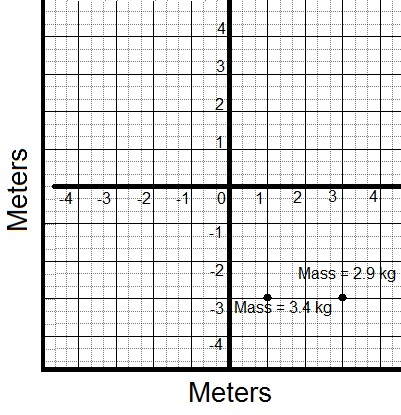# Finding the Center of Mass of a Group of Two or More Particles

with these practice questions

• 1.

In the following image a group of particles is shown. Find the center of mass.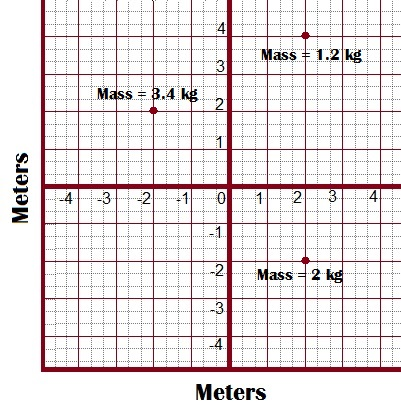• {eq}(\frac{2}{33},\frac{-38}{33}) {/eq}

• {eq}(\frac{-8}{32},\frac{5}{22}) {/eq}

• {eq}(\frac{8}{32},\frac{-5}{22}) {/eq}

• {eq}(\frac{-2}{33},\frac{38}{33}) {/eq}

• 2.

In the following graph a group of particles is shown calculate the center of mass.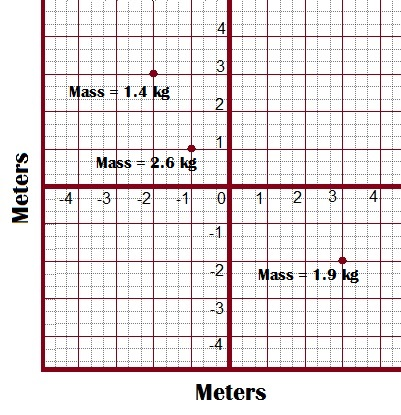• {eq}(\frac{-23}{59},\frac{-30}{59}) {/eq}

• {eq}(\frac{23}{59},\frac{-30}{59}) {/eq}

• {eq}(\frac{-3}{59},\frac{30}{59}) {/eq}

• {eq}(\frac{3}{59},\frac{30}{59}) {/eq}

• 3.

Calculate the center of mass of the group of particles shown in the next graph.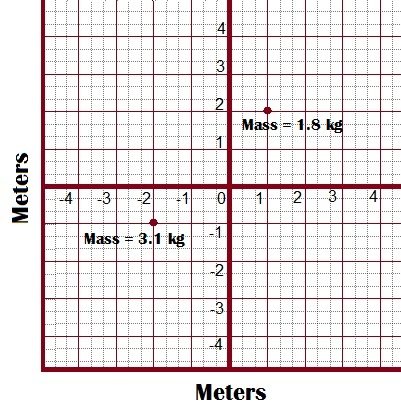• {eq}(\frac{35}{49},\frac{-5}{49}) {/eq}

• {eq}(\frac{-35}{49},\frac{5}{49}) {/eq}

• {eq}(\frac{-44}{49},\frac{5}{49}) {/eq}

• {eq}(\frac{44}{49},\frac{-5}{49}) {/eq}

• 4.

A group of particles is shown in the figure below. Determine the center of mass.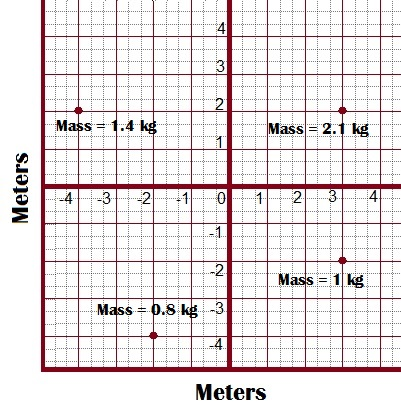• {eq}(\frac{-21}{53},\frac{26}{53}) {/eq}

• {eq}(\frac{-18}{53},\frac{33}{53}) {/eq}

• {eq}(\frac{18}{53},\frac{-33}{53}) {/eq}

• {eq}(\frac{21}{53},\frac{26}{53}) {/eq}

• 5.

In the graph we can observe a set of particles. The center of mass must be calculated.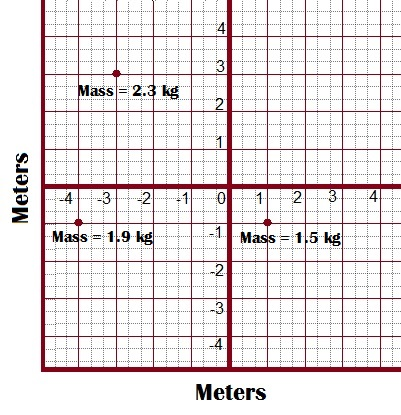• {eq}(\frac{120}{87},\frac{-35}{57}) {/eq}

• {eq}(\frac{-120}{87},\frac{35}{57}) {/eq}

• {eq}(\frac{-130}{57},\frac{35}{57}) {/eq}

• {eq}(\frac{130}{57},\frac{-35}{57}) {/eq}

• 6.

Calculate the center of mass in the group of particles that we can observe in the image below.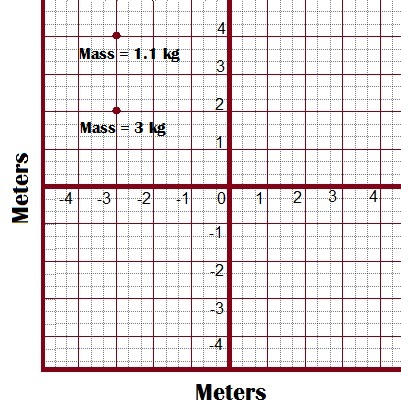• {eq}(-5,\frac{142}{41}) {/eq}

• {eq}(5,\frac{-142}{41}) {/eq}

• {eq}(3,\frac{-104}{41}) {/eq}

• {eq}(-3,\frac{104}{41}) {/eq}

• 7.

We must find the center of mass of the group of particles shown in the graph.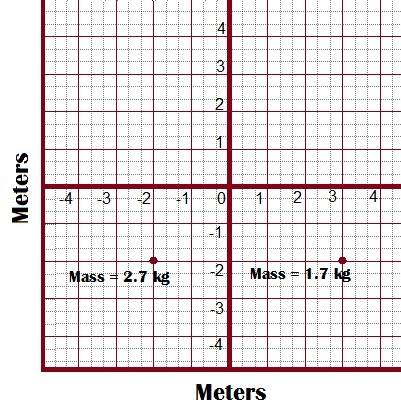• {eq}(\frac{4}{120},3) {/eq}

• {eq}(\frac{3}{44},2) {/eq}

• {eq}(\frac{-4}{120},-3) {/eq}

• {eq}(\frac{-3}{44},-2) {/eq}

• 8.

What is the center of mass of the particles found in this graph?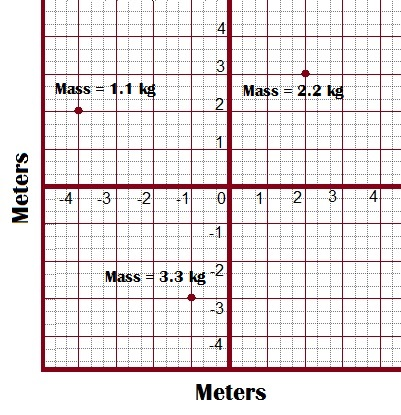• {eq}(\frac{-1}{2},\frac{-1}{6}) {/eq}

• {eq}(\frac{1}{2},\frac{1}{6}) {/eq}

• {eq}(\frac{-3}{2},\frac{-27}{100}) {/eq}

• {eq}(\frac{3}{2},\frac{27}{100}) {/eq}

• 9.

In the following image a group of particles is shown. What is the center of mass?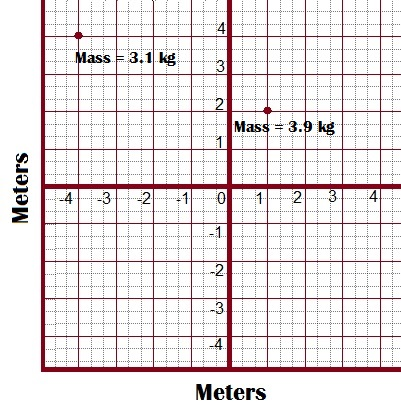• {eq}(\frac{-27}{14},\frac{111}{35}) {/eq}

• {eq}(\frac{-17}{14},\frac{101}{35}) {/eq}

• {eq}(\frac{17}{14},\frac{-101}{35}) {/eq}

• {eq}(\frac{27}{14},\frac{-111}{35}) {/eq}

• 10.

Calculate the center of mass of the group of particles shown in the next graph.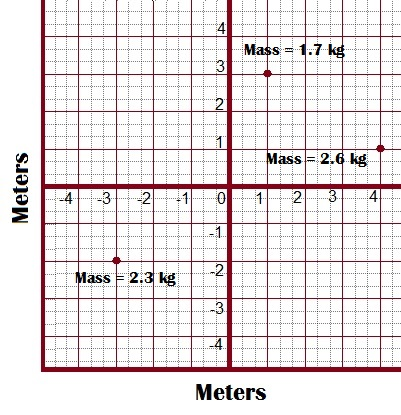• {eq}(\frac{79}{100},\frac{47}{100}) {/eq}

• {eq}(\frac{-79}{100},\frac{-47}{100}) {/eq}

• {eq}(\frac{47}{100},\frac{79}{100}) {/eq}

• {eq}(\frac{-47}{100},\frac{-79}{100}) {/eq}

• Become a Study.com member to unlock 20 more questions here and across thousands of other skills. Create your account

Put Student Mastery to the Test. Unlock Practice Questions Today!
Aligned with state standards.
• 11.

In the graph we can observe a set of particles. The center of mass must be calculated.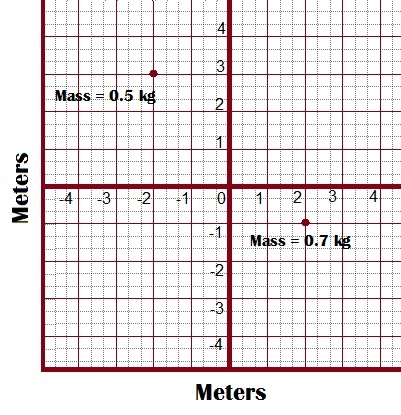• {eq}(\frac{-1}{3},\frac{-2}{3}) {/eq}

• {eq}(\frac{1}{3},\frac{2}{3}) {/eq}

• {eq}(\frac{33}{100},\frac{29}{12}) {/eq}

• {eq}(\frac{-33}{100},\frac{29}{12}) {/eq}

• 12.

We must find the center of mass of the group of particles shown in the graph.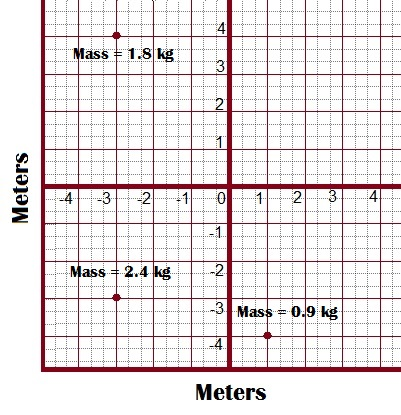• {eq}(\frac{39}{17},\frac{12}{17}) {/eq}

• {eq}(\frac{49}{17},\frac{9}{17}) {/eq}

• {eq}(\frac{-49}{17},\frac{-9}{17}) {/eq}

• {eq}(\frac{-39}{17},\frac{-12}{17}) {/eq}

• 13.

In the following image a group of particles is shown. Find the center of mass.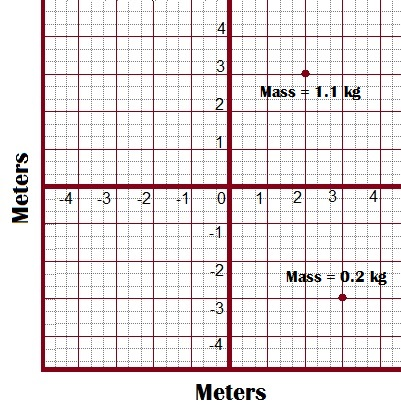• {eq}(\frac{28}{13},\frac{27}{13}) {/eq}

• {eq}(\frac{18}{13},\frac{17}{13}) {/eq}

• {eq}(\frac{27}{13},\frac{28}{13}) {/eq}

• {eq}(\frac{17}{13},\frac{18}{13}) {/eq}

• 14.

In the following image a group of particles is shown. What is the center of mass?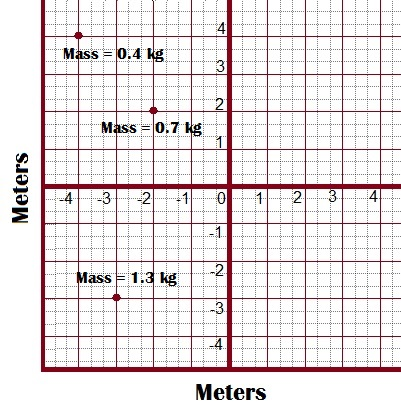• {eq}(\frac{-23}{8},\frac{3}{8}) {/eq}

• {eq}(\frac{-23}{8},\frac{-3}{8}) {/eq}

• {eq}(\frac{-13}{8},\frac{-21}{24}) {/eq}

• {eq}(\frac{-13}{8},\frac{21}{24}) {/eq}

• 15.

In the following graph a group of particles is shown. Calculate the center of mass.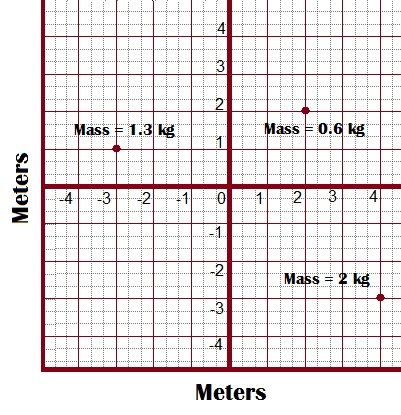• {eq}(\frac{-53}{39},\frac{-35}{39}) {/eq}

• {eq}(\frac{-23}{39},\frac{-25}{39}) {/eq}

• {eq}(\frac{23}{39},\frac{-25}{39}) {/eq}

• {eq}(\frac{53}{39},\frac{-35}{39}) {/eq}

• 16.

Calculate the center of mass of the group of particles shown in the following image.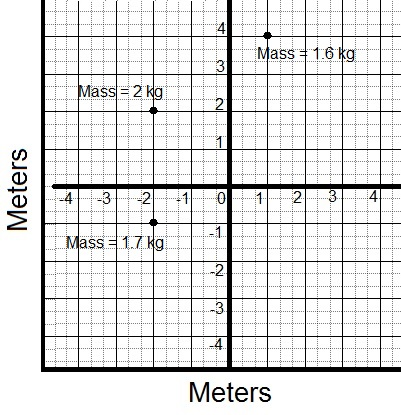• {eq}(\frac{-36}{33},\frac{-57}{33}) {/eq}

• {eq}(\frac{-58}{53},\frac{-87}{53}) {/eq}

• {eq}(\frac{-58}{53},\frac{87}{53}) {/eq}

• {eq}(\frac{-36}{33},\frac{57}{33}) {/eq}

• 17.

Determine the center of mass of the group of particles shown in the following image.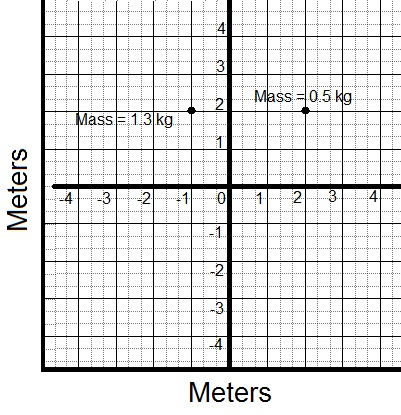• {eq}(\frac{5}{6},3) {/eq}

• {eq}(\frac{-1}{6},2) {/eq}

• {eq}(\frac{1}{6},2) {/eq}

• {eq}(\frac{-5}{6},3) {/eq}

• 18.

Find the center of mass of the group of particles shown in the image.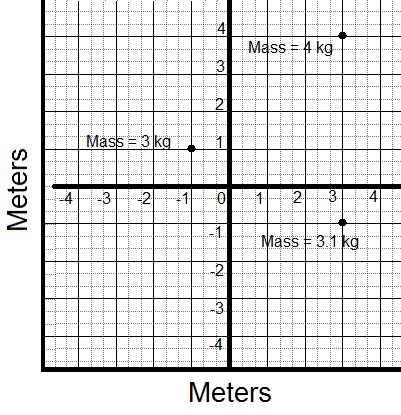• {eq}(\frac{83}{101},\frac{59}{101}) {/eq}

• {eq}(\frac{-83}{101},\frac{59}{101}) {/eq}

• {eq}(\frac{-183}{101},\frac{159}{101}) {/eq}

• {eq}(\frac{183}{101},\frac{159}{101}) {/eq}

• 19.

In the following image, calculate the center of mass of the group of particles shown.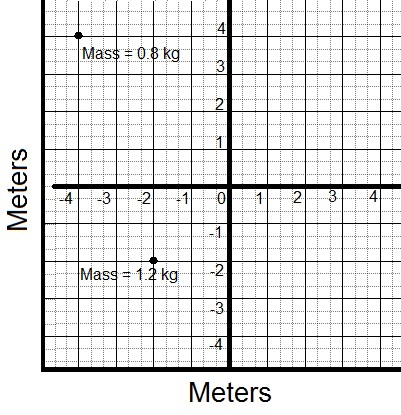• {eq}(\frac{14}{5},\frac{2}{5}) {/eq}

• {eq}(\frac{-14}{5},\frac{2}{5}) {/eq}

• {eq}(\frac{14}{5},\frac{-2}{5}) {/eq}

• {eq}(\frac{-14}{5},\frac{-2}{5}) {/eq}

• 20.

In this image, the center of mass of the group of particles shown must be calculated.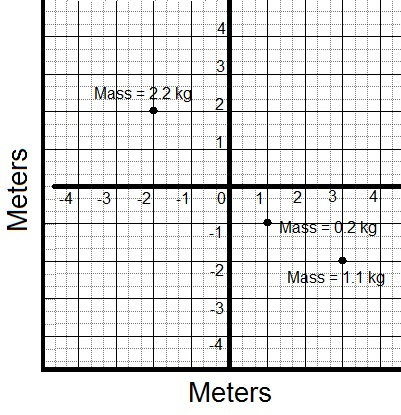• {eq}(\frac{-9}{35},\frac{4}{7}) {/eq}

• {eq}(\frac{9}{35},\frac{-4}{7}) {/eq}

• {eq}(\frac{-9}{35},\frac{-4}{7}) {/eq}

• {eq}(\frac{9}{35},\frac{4}{7}) {/eq}

• 21.

In the group of particles shown in this image, the center of mass must be determined.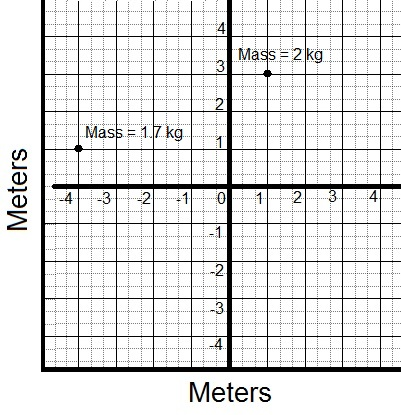• {eq}(\frac{48}{37},\frac{-77}{37}) {/eq}

• {eq}(\frac{-48}{37},\frac{77}{37}) {/eq}

• {eq}(\frac{-48}{37},\frac{-77}{37}) {/eq}

• {eq}(\frac{48}{37},\frac{77}{37}) {/eq}

• 22.

The following image represents a group of particles in which the center of mass must be found.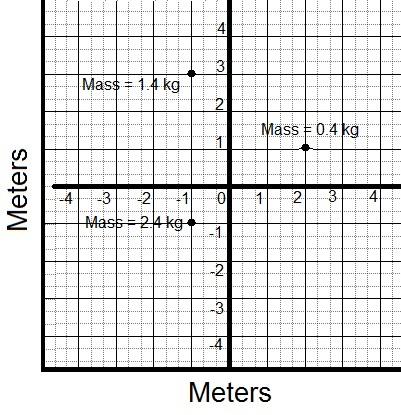• {eq}(\frac{-5}{7},\frac{-11}{21}) {/eq}

• {eq}(\frac{-5}{7},\frac{11}{21}) {/eq}

• {eq}(\frac{5}{7},\frac{-11}{21}) {/eq}

• {eq}(\frac{5}{7},\frac{11}{21}) {/eq}

• 23.

The table below shows a group of particles in which the center of mass is to be calculated.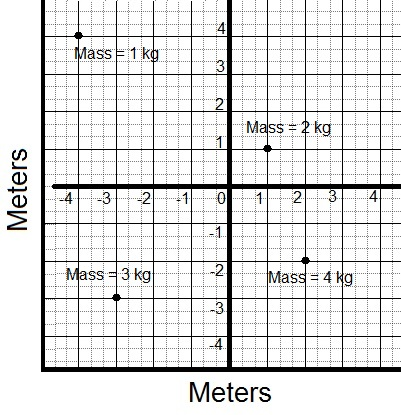• {eq}(\frac{-3}{10},\frac{11}{10}) {/eq}

• {eq}(\frac{-3}{10},\frac{-11}{10}) {/eq}

• {eq}(\frac{3}{10},\frac{-11}{10}) {/eq}

• {eq}(\frac{3}{10},\frac{11}{10}) {/eq}

• 24.

Calculate the center of mass of the group of particles shown in the following image.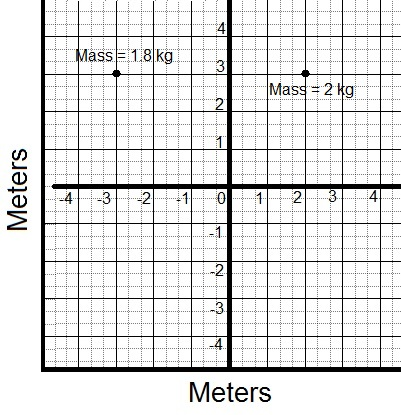• {eq}(\frac{7}{19},-3) {/eq}

• {eq}(\frac{-7}{19},-3) {/eq}

• {eq}(\frac{7}{19},3) {/eq}

• {eq}(\frac{-7}{19},3) {/eq}

• 25.

In the following image, calculate the center of mass of the group of particles shown.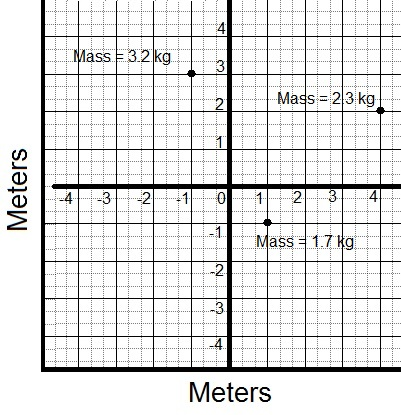• {eq}(\frac{-77}{72},\frac{125}{72}) {/eq}

• {eq}(\frac{77}{72},\frac{125}{72}) {/eq}

• {eq}(\frac{77}{72},\frac{-125}{72}) {/eq}

• {eq}(\frac{-77}{72},\frac{-125}{72}) {/eq}

• 26.

The following image represents a group of particles in which the center of mass must be found.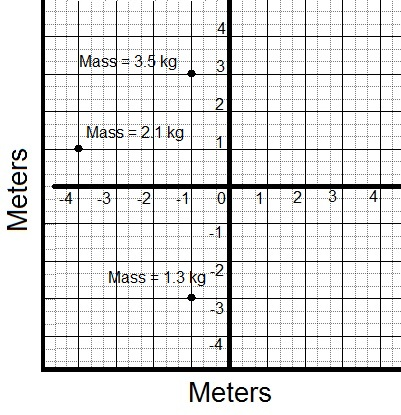• {eq}(\frac{44}{23},\frac{29}{23}) {/eq}

• {eq}(\frac{44}{23},\frac{-29}{23}) {/eq}

• {eq}(\frac{-44}{23},\frac{-29}{23}) {/eq}

• {eq}(\frac{-44}{23},\frac{29}{23}) {/eq}

• 27.

Determine the center of mass of the group of particles shown in the following image.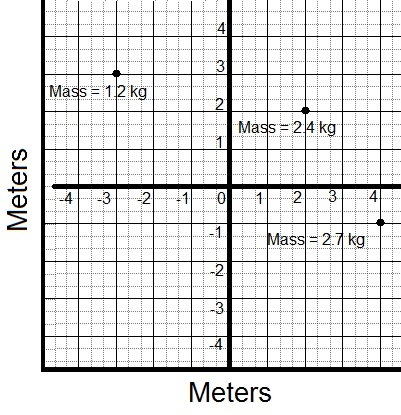• {eq}(\frac{-40}{21},\frac{-19}{21}) {/eq}

• {eq}(\frac{19}{21},\frac{-37}{21}) {/eq}

• {eq}(\frac{40}{21},\frac{19}{21}) {/eq}

• {eq}(\frac{40}{21},\frac{-19}{21}) {/eq}

• 28.

In this image, the center of mass of the group of particles shown must be calculated.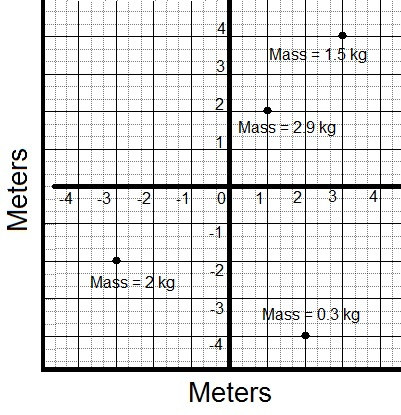• {eq}(\frac{-20}{67},\frac{66}{67}) {/eq}

• {eq}(\frac{-20}{67},\frac{-66}{67}) {/eq}

• {eq}(\frac{20}{67},\frac{66}{67}) {/eq}

• {eq}(\frac{20}{67},\frac{-66}{67}) {/eq}

• 29.

Calculate the center of mass of the group of particles shown in the following image.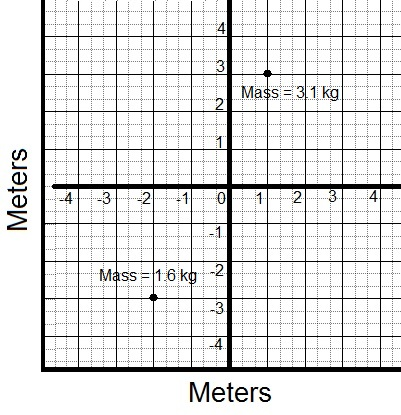• {eq}(\frac{-1}{47},\frac{-45}{47}) {/eq}

• {eq}(\frac{1}{47},\frac{-45}{47}) {/eq}

• {eq}(\frac{-1}{47},\frac{45}{47}) {/eq}

• {eq}(\frac{1}{47},\frac{45}{47}) {/eq}

• 30.

Determine the center of mass of the group of particles shown in the following image.